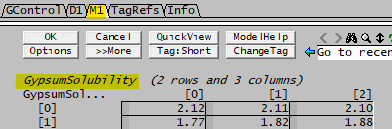# Table lookup using Array and Matrix

Navigation: PGMs ➔ Example PGM Files ➔ Lookup Value

Simple Examples Subroutines Examples Dynamic Examples Steady State Overall Mass Balance Array and Matrix Examples
Basic
Layout
Simple
Calculations
Initialise
PreStart
Multi-Step Trigger Checking
Project
Counter, While
and Random
Belt Filter
Wash Loss
Startup
Actions
Mass
Balance
Mass
Balance
Species
Balance
Elemental
Balance
Lookup
Value
Set
Values
Tridiagonal
System

Related Links: PGM Files using Class and Functions, Matrix Class, Array Class

## PGM FILE - Look up Gypsum Solubility from a Table using SysCAD 9.3 Syntax

This example shows how to look up the Gypsum solubility from a table based on Temperature and acid concentration.

A example table may look like this, note this data is for PGM demonstration purpose only, please use with caution.

 Gypsum Solubility (g/L) Temperature (C) Acid Conc (g/L) 25 30 35 0 2.12 2.11 2.10 9.8 1.77 1.82 1.88

If we have a solution at 28 dC, and acid concentration at 5 g/L, we want to look up the gypsum solubility from the above table.

• The above table only gives temperature range in 5 deg increments, so we will use the 30 dC column. At 30 dC, we have values for gypsum solubility for acid concentration of 0 g/L and 9.8 g/L. We will use linear interpolation to obtain the solubility value at acid concentration at 5 g/L.
• The result is therefore 2.11 + (1.82 - 2.11) * (5 - 0)/(9.8-0) = 1.96 g/L

We can define the table values directly into the PGM file, then look up the values based on the current conditions:

1. Create two arrays with constant values, to represent row heading (acid concentration) and column heading (temperature segments)
2. Create a matrix with constant values to represent the Gypsum solubility values.
3. Match the current/required temperature to the nearest temperature segment
4. Match the current/required acid concentration to obtain the gypsum solubility values from the table.
5. Perform linear interpolation to obtain the gypsum solubility.

EXAMPLE PGM file that does the above is listed below:

;--- SysCAD General Controller (PGM) file ---
; Revision: 2     Date:  Jan 2023   Author: SysCAD Team

;--- variable declarations ---

REAL    CurrentTemp@("T", "C"), TemperatureSegment@("T", "C")
REAL    CurrentAcidConc@("Conc", "g/L"), AcidConcPt1@("Conc", "g/L"), AcidConcPt2@("Conc", "g/L")
REAL    GypsumSol@("Conc", "g/L"), GypsumSolPt1@("Conc", "g/L"), GypsumSolPt2@("Conc", "g/L")

const Array  Temperature = {25, 30, 35}
const Array  AcidConc = {0, 9.8}
const matrix GypsumSolubility = { {2.12, 2.11, 2.10}, {1.77, 1.82, 1.88} }

;Array	Temperature = {25, 30, 35}                                     ;Old syntax before Build 139.32325
;Array	AcidConc = {0, 9.8}                                            ;Old syntax before Build 139.32325
;matrix  GypsumSolubility = { {2.12, 2.11, 2.10}, {1.77, 1.82, 1.88} } ;Old syntax before Build 139.32325

Watch GypsumSolubility

LONG    RowCount@, ColCount@, TemperatureIndex@, AcidIndex@
BYTE    i, j

REAL    modTemp, temp, temp1

Sub InitialiseSolution()
RowCount = GypsumSolubility.GetRowCount()
ColCount = GypsumSolubility.GetColCount()
endSub

; Get the current temperature and round it to the closest value of multiple 5
CurrentTemp = ["P_003.To (C)"]
modTemp = MOD(CurrentTemp, 5)
TemperatureSegment= IIF(modTemp>=2.500001, ROUND(CurrentTemp+(5-modTemp)), ROUND(CurrentTemp-modTemp))
;limits the temperature to the maximum T defined in the array
TemperatureSegment= MIN(Temperature[ColCount-1], TemperatureSegment)

;Match the current temperature to the temperature index number (column number)
i = 0
While i < ColCount
temp = Temperature[i]
If temp == TemperatureSegment
TemperatureIndex = i
i=ColCount
endif
i = i + 1
Endwhile

;Match the current acid concentration to the acid index number (row number)
CurrentAcidConc = ["P_003.Qo.CMC:LPh.H2SO4 (g/L)"]
j = 0
While j < RowCount
temp1 = AcidConc[j]
If temp1 >= CurrentAcidConc
AcidIndex = j
j=RowCount
endif
j = j + 1
Endwhile

;Get Gypsum Solubility using the Temperature and acid Index, and apply interpolation
GypsumSolPt1 = GypsumSolubility.GetAt(AcidIndex-1, TemperatureIndex)
GypsumSolPt2 = GypsumSolubility.GetAt(AcidIndex, TemperatureIndex)

AcidConcPt1 = AcidConc[AcidIndex-1]
AcidConcPt2 = AcidConc[AcidIndex]

GypsumSol = GypsumSolPt1 + (GypsumSolPt2 - GypsumSolPt1) * ((CurrentAcidConc - AcidConcPt1)/(AcidConcPt2-AcidConcPt1))

\$  ; --- end of file ---